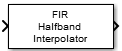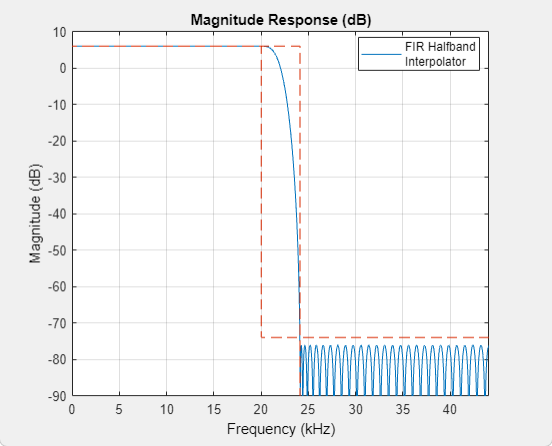# FIR Halfband Interpolator

Interpolate signal using polyphase FIR half band filter

## Library

Filtering / Filter Designs

`dspfdesign`

•## Description

The FIR Halfband Interpolator block performs interpolation of the input signal by a factor of two. The block uses an FIR equiripple design to construct the halfband filters. To filter the input, the block uses an efficient polyphase implementation. The implementation takes advantage of the zero-valued coefficients of the FIR halfband filter, making one of the polyphase branches a delay. You can also use the block to implement the synthesis portion of a two-band filter bank to synthesize a signal from lowpass and highpass subbands.

The input signal can be a real- or complex-valued column vector or matrix. If the input signal is a matrix, each column of the matrix is treated as an independent channel. The block supports fixed-point operations and ARM® Cortex® code generation. For more information on ARM Cortex code generation, refer Code Generation for ARM Cortex-M and ARM Cortex-A Processors.

## Dialog Box

### Main Tab

Filter specification

Parameters used to design the FIR halfband filter.

• ```Transition width and stopband attenuation``` (default) — Design the filter using Transition width (Hz) and Stopband attenuation (dB). This design is the minimum order design.

• ```Filter order and transition width``` — Design the filter using Filter order and Transition width (Hz).

• ```Filter order and stopband attenuation``` — Design the filter using Filter order and Stopband attenuation (dB).

• `Coefficients` — Specify the filter coefficients directly through the Numerator parameter.

Transition width (Hz)

Transition width, specified as a real positive scalar in Hz. The transition width must be less than half the input sample rate. You can specify the transition width only when Filter specification is set to ```Filter order and transition width``` or ```Transition width and stopband attenuation```. The default is `4.1e3`.

Filter order

Filter order, specified as an even positive integer. You can specify only when Filter specification is set to ```Filter order and transition width``` or ```Filter order and stopband attenuation```. The default is `52`.

Stopband attenuation (dB)

Stopband attenuation, specified as a real positive scalar in dB. You can specify the stopband attenuation only when Filter specification is set to ```Filter order and stopband attenuation``` or ```Transition width and stopband attenuation```. The default is `80`.

Numerator

Specify the FIR halfband filter coefficients directly as a row vector. The coefficients must comply with the FIR halfband impulse response format. If half the order of the filter, `(length(Numerator) - 1)/2`, is even, every other coefficient starting from the first coefficient must be a zero except for the center coefficient, which must be a 1.0. If half the order of the filter is odd, the sequence of alternating zeros with a 1.0 at the center starts at the second coefficient.

This parameter appears when Filter specification is set to `'Coefficients'`. The default is the coefficients vector returned by `2*firhalfband('minorder',0.407,1e-4)`.

Input highpass subband

When you select this check box, the block acts as a synthesis filter bank and synthesizes a signal from the highpass and lowpass subbands. When you clear this check box, the block acts as an FIR half band interpolator and accepts a single vector– or matrix–valued input.

Inherit sample rate from input

When you select this check box, the block inherits its sample rate from the input signal. When you clear this check box, you specify the sample rate in Input Sample Rate (Hz). This parameter appears when you set Filter specification to any option other than `Coefficients`.

Input sample rate (Hz)

Input sample rate, specified as a scalar in Hz. The default value is `44100`. This parameter appears when you set Filter specification to any option other than `Coefficients` and clear the Inherit sample rate from input parameter.

View Filter Response

Opens the Filter Visualization Tool FVTool and displays the magnitude/phase response of the FIR Halfband Interpolator. The response is based on the block dialog box parameters. Changes made to these parameters update FVTool.To update the magnitude response while FVTool is running, modify the dialog box parameters and click .

Simulate using

Type of simulation to run. You can set this parameter to:

• `Code generation` (default)

Simulate model using generated C code. The first time you run a simulation, Simulink® generates C code for the block. The C code is reused for subsequent simulations, as long as the model does not change. This option requires additional startup time but provides faster simulation speed than ```Interpreted execution```.

• ```Interpreted execution```

Simulate model using the MATLAB®  interpreter. This option shortens startup time but has slower simulation speed than ```Code generation```.

### Data Types Tab

Rounding mode

Rounding method for the output fixed-point operations. The rounding methods are `Ceiling`, `Convergent`, `Floor`, `Nearest`, `Round`, `Simplest`, and `Zero`. The default is `Floor`.

Coefficients

Fixed-point data type of the coefficients, specified as one of the following:

• `fixdt(1,16)` (default) — Signed fixed-point data type of word length `16`, with binary point scaling. The block determines the fraction length automatically from the coefficient values in such a way that the coefficients occupy maximum representable range without overflowing.

• `fixdt(1,16,0)` — Signed fixed-point data type of word length `16` and fraction length, `0`. You can change the fraction length to any other integer value.

• ```<data type expression>``` — Specify the data type using an expression that evaluates to a data type object, for example, numeric type (`[ ]`,`16`, `15`). Specify the sign mode of this data type as `[ ]` or `true`.

• `Refresh Data Type` — Refreshes to the default data type.

Click the buttonto display the data type assistant, which helps you set the stage input parameter.

## Supported Data Types

PortSupported Data Types

Input

• Double-precision floating point

• Single-precision floating point

• Fixed point (signed or unsigned)

• 8-, 16-, 32-, and 64-bit signed integers

• real and complex data

Output

• Double-precision floating point

• Single-precision floating point

• Fixed point (signed only)

• 8-, 16-, 32-, and 64-bit signed integers

• real and complex data

 `dsp.FIRHalfbandInterpolator` DSP System Toolbox `dsp.FIRHalfbandDecimator` DSP System Toolbox FIR Halfband Decimator DSP System Toolbox

## Algorithms

This block brings the capabilities of the `dsp.FIRHalfbandInterpolator` System object™ to the Simulink environment.

For information on the algorithms used by this block, see the Algorithms section of `dsp.FIRHalfbandInterpolator`.

Watch now